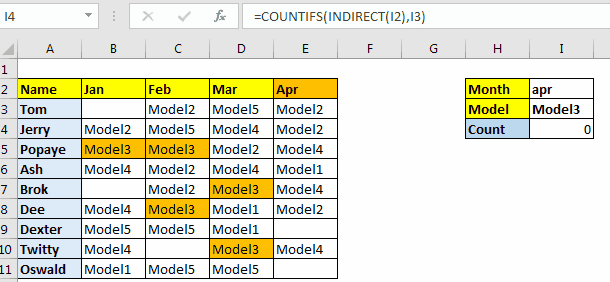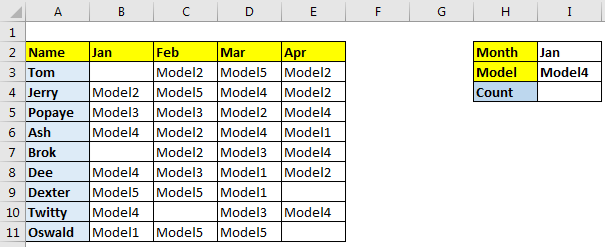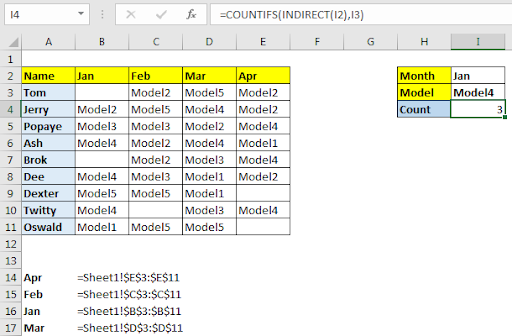# Get the COUNTIFS with Dynamic Criteria Range in ExcelWe know that COUNTIFS function in Excel can count on multiple criterias. It takes arguments as couple of criteria range and criteria. We can change criteria dynamically by giving the reference of cell but we can’t change the criteria column dynamically. Well, not directly but we can. That’s what we learn in advance excel formulas. Doing things in excel which can’t be done normally. Let’s see how.

Let’s learn by example.

Context
Here I have prepared a data of sales done in different months of year by our sales consultants. They sales different models of our product, generically named model1, model2 and so on. What we need to do is to count sales of different models in different months dynamically.In cell I2 we will choose the month. In cell I2 we will choose the model. These values can be will change. And the count should change too. The COUNTIFS Function should look for the month column that will be criteria range. Then it will look for model in that months column.
So here the criteria and criteria_range both are variable. So how do we make column variable in COUNTIFS? Here is how?
Using Named Range For Variable Column or Criteria Range
Generic Formula

=COUNTIFS(INDIRECT(named_range),criteria)

First, name each column as per their headings. To do so select the table and press CTRL+SHIFT+F3 and name the columns as per top row. Read about it here.
So, Range B3:B11, C3:C11, D3:D11, and E3:E11 are named Jan, Feb, Mar and Apr respectively.
Write this formula in I4.Now if you change the month in I4 the respective months count of Model4 will be shown in I4.
How It Works?
The formula is simple. Let’s start from inside.
INDIRECT(I2): As we know INDIRECT function converts text ref to actual reference. We have provided it I2. I2 contains Apr. Since we have range E3:E11 name Apr, INDIRECT(I2) translates to E3:E11.

The formula simplified to =COUNTIFS(E3:E11,I3). COUNTIFS counts whatever is in I3 in range E3:E11.

When you change month the column will change dynamically. This is called COUNTIFS with Variable columns. In the gif I have used some conditional formatting based on another cell.

The formula can also work with countif function. But if you want to have multiple conditions then use COUNTIFS function.

Related Article:

Creative Column Chart that Includes Totals

Create Overlay Chart in Excel 2016

Excel Sparklines : The Tiny Charts in Cell

Speedometer (Gauge) Chart in Excel 2016

Terms and Conditions of use

The applications/code on this site are distributed as is and without warranties or liability. In no event shall the owner of the copyrights, or the authors of the applications/code be liable for any loss of profit, any problems or any damage resulting from the use or evaluation of the applications/code.